﻿ 剪张源约束下的单井微地震震源机制反演
 石油地球物理勘探2021, Vol. 56Issue (5): 1093-1104  DOI: 10.13810/j.cnki.issn.1000-7210.2021.05.0160文章快速检索 高级检索

### 引用本文LIU Lei, SONG Weiqi, YANG Xiaohui, HU Jianlin, DONG Lin, YU Zhichao. Single-well microseismic focal mechanism inversion under shear-tensile source constraint. Oil Geophysical Prospecting, 2021, 56(5): 1093-1104. DOI: 10.13810/j.cnki.issn.1000-7210.2021.05.016.### 作者简介宋维琪, 山东省青岛市黄岛区长江西路66号中国石油大学(华东)地球科学与技术学院, 266580。Email: swq1123@126.com

### 文章历史

1 中国石油大学(华东)地球科学与技术学院, 山东青岛 266580;
2 中国石化石油物探技术研究院, 江苏南京 211103;
3 北京大学地球与空间科学学院石油与天然气研究中心, 北京 100871

Single-well microseismic focal mechanism inversion under shear-tensile source constraint
LIU Lei1 , SONG Weiqi1, YANG Xiaohui2 , HU Jianlin1 , DONG Lin1 , YU Zhichao3
1 School of Geosciences, China University of Petro-leum(East China), Qingdao, Shandong 266580, China;
2 SINOPEC Geophysical Research Institute, Nanjing, Jiangsu 211103, China;
3 Institute of Oil & Gas, School of Earth and Space Sciences, Peking University, Beijing 100871, China
Abstract: The microseismic monitoring data of hydraulic fracturing contain the information of focal mechanism, which can be used to describe the fracture type and stress state of the working area and is thus of great significance to the evaluation of fracturing effect and the guidance of subsequent fracturing scheme. Due to the small monitoring angle and single measure direction, full moment tensor inversion is hard to perform in single-well observation systems. In this study, comprehensively utilizing fracturing holes, logging and location data, we design a target function based on P- and S-wave energy and first-arrival polarity matching from three-component microseismic recordings under the constraint of the focus to shear-tensile dislocation model. Further, we realize the single-well microseismic focal mechanism inversion with full-space grid search. Synthetic data test shows that the inversion method has good accuracy and noise resis-tance. Specifically, the inversion accuracy of each parameter is more than 81% under the noise-free condition and is still higher than 51% in the pre-sence of 10dB noise. The anti-noise abilities of different parameters are in the order of tensile angle>dip angle>strike angle>slide angle. Finally, we apply this method to actual microseismic data of shale fracturing. The inversion results show that microseismic events of the fracturing are mainly formed by rock rupture and slip caused by one pair of couple or two pairs with different magnitudes, and the anti-tensile fracture formed by compression is more than tensile fracture formed by expansion.
Keywords: borehole microseismic event    focal mechanism    shear-tensile dislocation model    moment tensor
0 引言

1 方法原理 1.1 矩张量与剪张源张量

 $\boldsymbol{M}=M_{0}\left[\begin{array}{lll} M_{11} & M_{12} & M_{13} \\ M_{21} & M_{22} & M_{23} \\ M_{31} & M_{32} & M_{33} \end{array}\right]$ (1)

 $\left\{\begin{array}{l} n_{1}=-\sin \delta \sin \varphi \\ n_{2}=\sin \delta \cos \varphi \\ n_{3}=-\cos \delta \end{array}\right.$ (2)
 \left\{\begin{aligned} v_{1}=&(\cos \psi \cos \varphi+\cos \delta \sin \psi \sin \varphi) \cos \alpha-\\ & \sin \delta \sin \varphi \sin \alpha \\ v_{2}=&(\cos \psi \sin \varphi-\cos \delta \sin \psi \cos \varphi) \cos \alpha+\\ & \sin \delta \cos \varphi \sin \alpha \\ v_{3}=&-\sin \psi \sin \delta \cos \alpha-\cos \delta \sin \alpha \end{aligned}\right. (3)
 \begin{aligned} &\boldsymbol{D}=\frac{T}{2}\left(\boldsymbol{n}^{\mathrm{T}} \boldsymbol{v}+\boldsymbol{v}^{\mathrm{T}} \boldsymbol{n}\right) \\ &=\frac{T}{2}\left[\begin{array}{ccc} 2 n_{1} v_{1} & n_{1} v_{2}+n_{2} v_{1} & n_{1} v_{3}+n_{3} v_{1} \\ n_{1} v_{2}+n_{2} v_{1} & 2 n_{2} v_{2} & n_{2} v_{3}+n_{3} v_{2} \\ n_{1} v_{3}+n_{3} v_{1} & n_{2} v_{3}+n_{3} v_{2} & 2 n_{3} v_{3} \end{array}\right] \end{aligned} (4)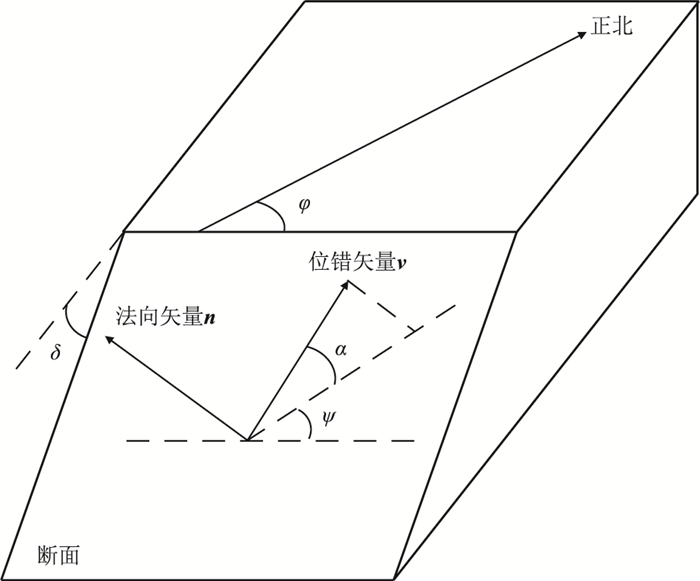图 1 剪切—张裂源模型示意图

 $M_{i j}=c_{i j k l} D_{k l}$ (5)

 $M_{i j}=\lambda D_{k k} \delta_{i j}+2 \mu D_{i j}$ (6)

1.2 矩张量微地震波场记录正演

Aki等将各向同性介质中矩张量震源下的远波场表示为

 \begin{aligned} u_{i}(\boldsymbol{x}, t)=& \frac{R_{i}^{\mathrm{P}} f\left(t-\tau^{\mathrm{P}}\right)}{4 {\rm{ \mathsf{ π} }} \rho r A^{3}}+\frac{R_{i}^{\mathrm{SH}} f\left(t-\tau^{\mathrm{SH}}\right)}{4 {\rm{ \mathsf{ π} }} \rho r B_{\mathrm{SH}}^{3}}+\\ & \frac{R_{i}^{\mathrm{SV}} f\left(t-\tau^{\mathrm{SV}}\right)}{4 {\rm{ \mathsf{ π} }} \rho r B_{\mathrm{SV}}^{3}} \end{aligned} (7)

 $R_{i}^{\mathrm{P}}=m_{i} m_{j} m_{k} M_{j k}$ (8)
 $R_{i}^{\mathrm{S}}=\delta_{i j} m_{k} M_{i j}-R_{i}^{\mathrm{P}}$ (9)
 $R_{i}^{\mathrm{SV}} =q_{i} q_{j} R_{i}^{\mathrm{S}}$ (10)
 $R_{i}^{\mathrm{SH}} =o_{i} o_{j} R_{j}^{\mathrm{S}}$ (11)

 $\left\{\begin{array}{l} \boldsymbol{m}=(\sin \beta \cos \theta, \sin \beta \sin \theta, \cos \beta) \\ \boldsymbol{q}=(\cos \beta \cos \theta, \cos \beta \sin \theta,-\sin \beta) \\ \boldsymbol{o}=(-\sin \theta, \cos \theta, 0) \end{array}\right.$ (12)

1.3 基于波形能量和极性的震源机制反演方法

(1) 通过微地震监测资料、测井资料和射孔资料等获得微地震事件波形记录，及其对应的震源位置、观测点位置、地层速度模型等信息。

(2) 井中微地震资料偏振归位处理。根据射孔资料，震源和检波器相对位置信息进行偏振角的计算，并进行偏振处理实现三分量记录中水平分量波形记录旋转为观测坐标系统下的xy分量。

(3) 利用微地震事件P、S波初至选取有效时窗长度k。实际微地震事件能量大小不一导致振幅数据不一致，为消除震级大小影响，需要对事件进行振幅归一化。计算振幅归一化后波形窗口内P、S波能量EIPEIS

 $\left\{\begin{array}{l} E_{I}^{\mathrm{P}}=\sum\limits_{t=t_{\mathrm{P}}}^{t_{\mathrm{P}}+k_{\mathrm{P}}} W_{I}^{2}(t) \\ E_{I}^{\mathrm{S}}=\sum\limits_{t=t_{\mathrm{S}}}^{t_{\mathrm{S}}+k_{\mathrm{S}}} W_{I}^{2}(t) \end{array}\right.$ (13)

(4) 实际微地震事件波形初至极性PI识别和主频分析。初至极性为正极性记为1，负极性记为-1，正演时子波采用雷克子波对时间的偏导数，该函数的初至为负值，因此实测资料中初至为负值时表现为正极性，初至为正值时表现为负极性；主频会影响子波的“胖瘦”程度，从而影响能量计算，而且实际井中观测的微地震事件P、S波主频不完全一致，有时会有较大差别而造成反演误差。

(5) 在不同走向角φ、倾角δ、滑动角ψ和张裂角α网格剖分下正演的波场记录后，根据步骤(3)、步骤(4)求取该网格点模型正演的P、S波波场能量eIPeIS以及初动极性pn，设计目标函数

 \begin{aligned} F=& \sum\limits_{I=1}^{N} \sum\limits_{i=1}^{3}\left(a_{1}\left|E_{i, I}^{\mathrm{P}}-e_{i, I}^{\mathrm{P}}\right|+\right.\\ &\left.a_{2}\left|E_{i, I}^{\mathrm{S}}-e_{i, I}^{\mathrm{S}}\right|+a_{3}\left|P_{i, I}-p_{i, I}\right|\right) \end{aligned} (14)

(6) 选取一定量极小目标函数网格点对应解，并继续向下剖分，重复步骤(5)，直至满足求解精度要求。

2 模型测试 2.1 模型正、反演表 1 速度模型参数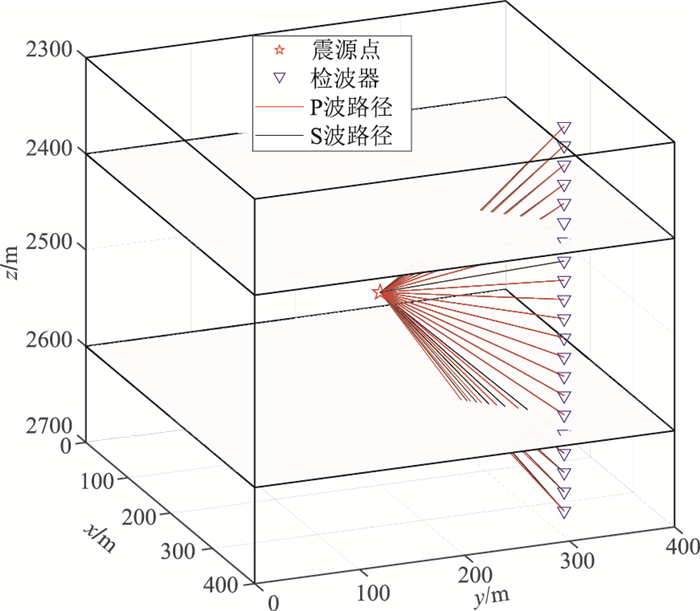图 2 模型及观测系统表 2 剪张型模拟震源的参数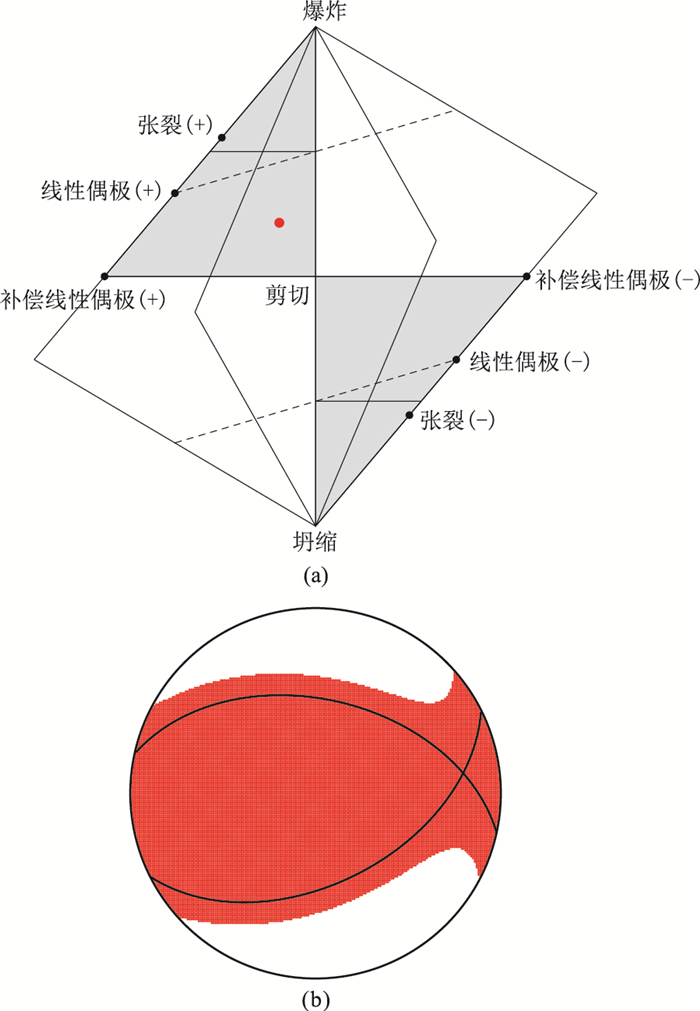图 3 剪张型震源机制的Hudson投影(a)及沙滩球表示(b)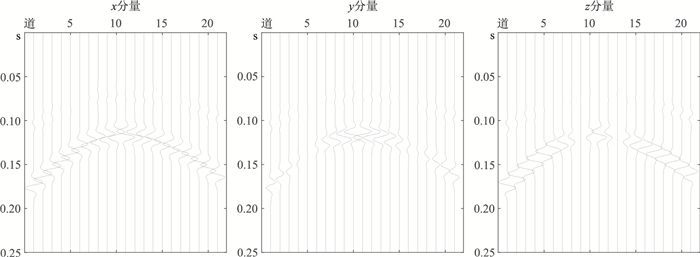图 4 三层水平层状介质正演三分量微地震事件透射波波形记录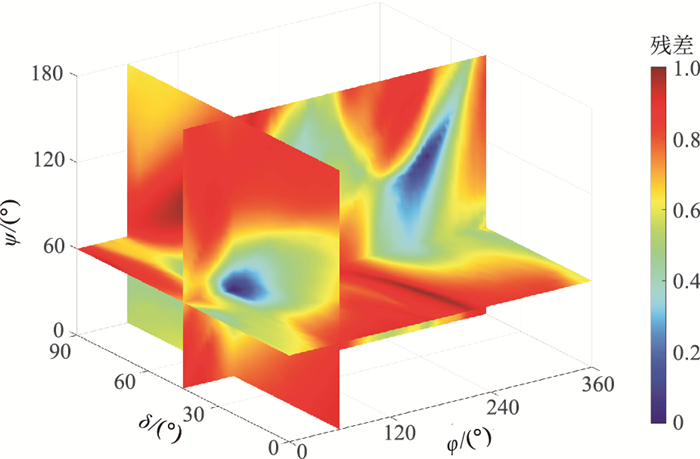图 5 模拟数据反演目标函数残差在走向角、倾向角和滑动角三参数的全网格分布
2.2 无噪声数据反演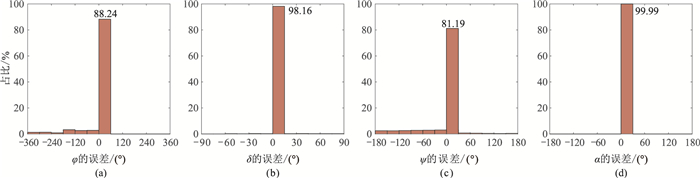图 6 无噪声情况下走向角(a)、倾向角(b)、滑动角(c)和张裂角(d)的反演误差统计

2.3 含噪声数据及扰动速度模型反演

 $\mathrm{SNR}=20 \lg \frac{\|S\|_{F}}{\|\xi\|_{F}}$ (15)表 3 扰动速度模型参数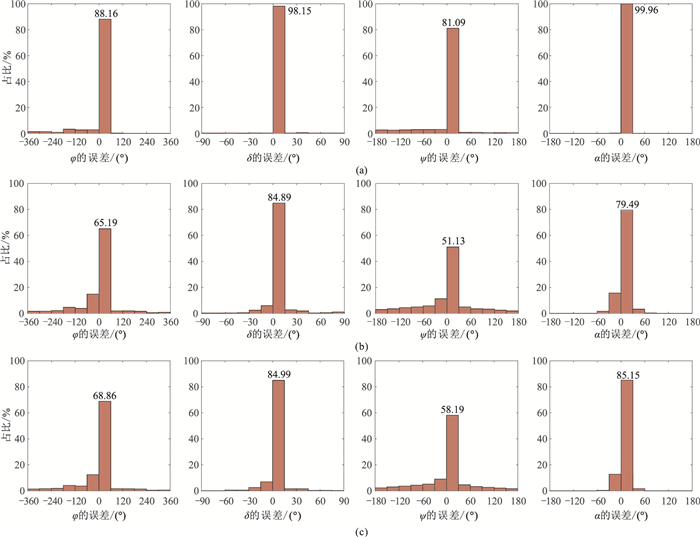图 7 不同信噪比及扰动速度模型情况下“剪张型”震源机制的四个参数反演误差统计 (a)40dB信噪比；(b)10dB信噪比；(c)速度模型扰动10%
3 实际压裂资料震源机制反演 3.1 实测资料预处理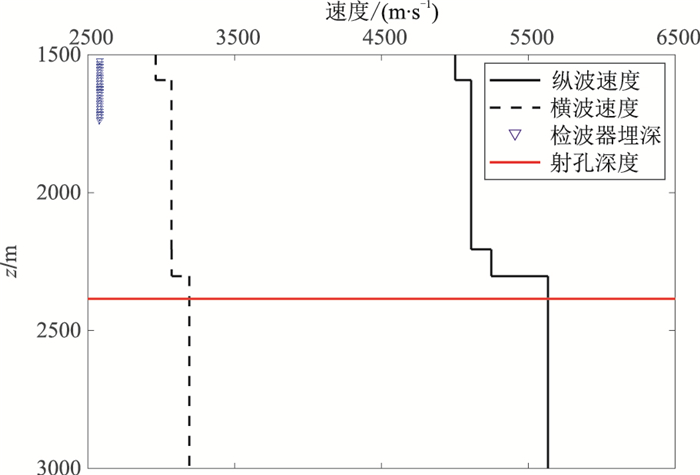图 8 工区速度模型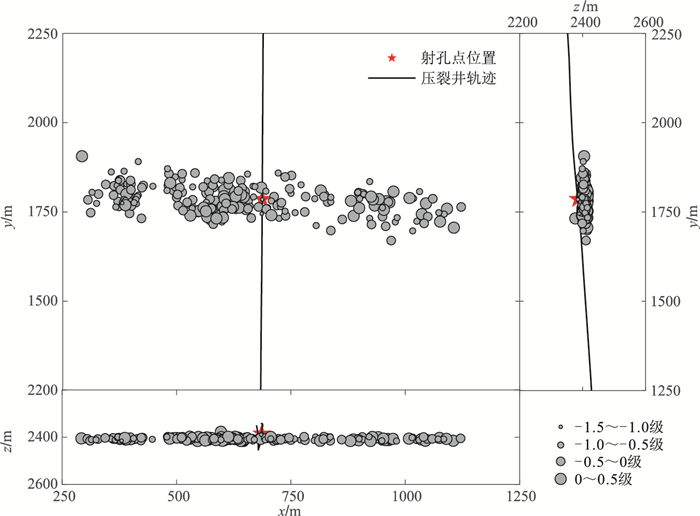图 9 水力压裂微地震震源定位结果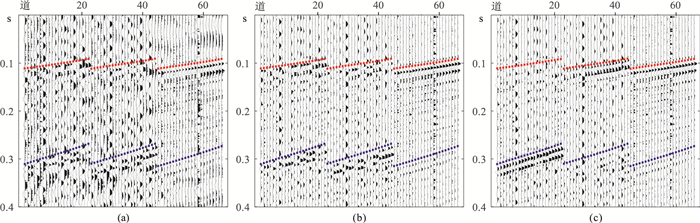图 10 实际三分量监测资料及预处理结果 (a)原始记录；(b)带通滤波记录；(c)水平分量旋转记录。1~22道为x分量；23~44道为y分量；45~66道为z分量 红色和蓝色虚线分别表示手动拾取的P和S波初至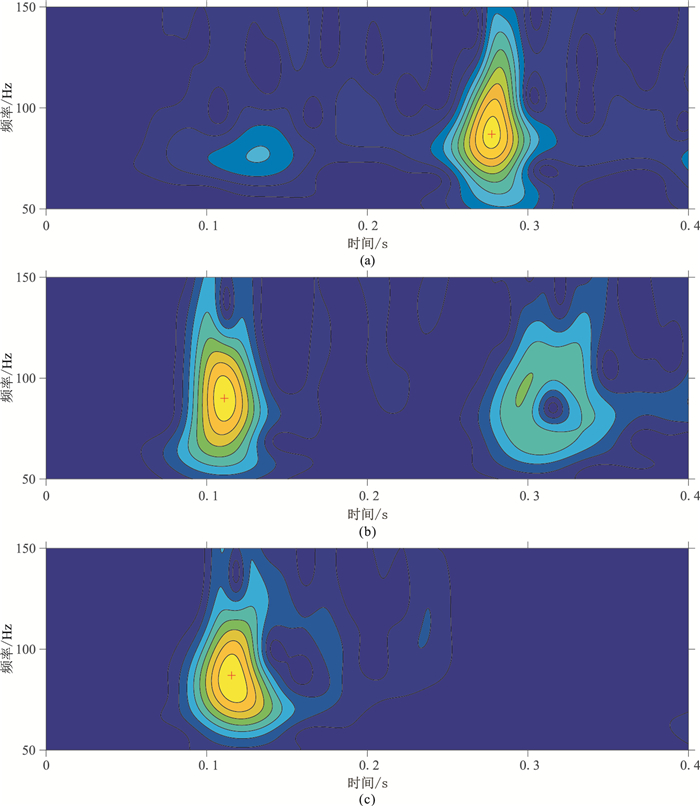图 11 三分量微地震记录时频率分析结果 (a)x分量；(b)y分量；(c)z分量。红色“+”标示初至主频位置
3.2 实测资料震源机制反演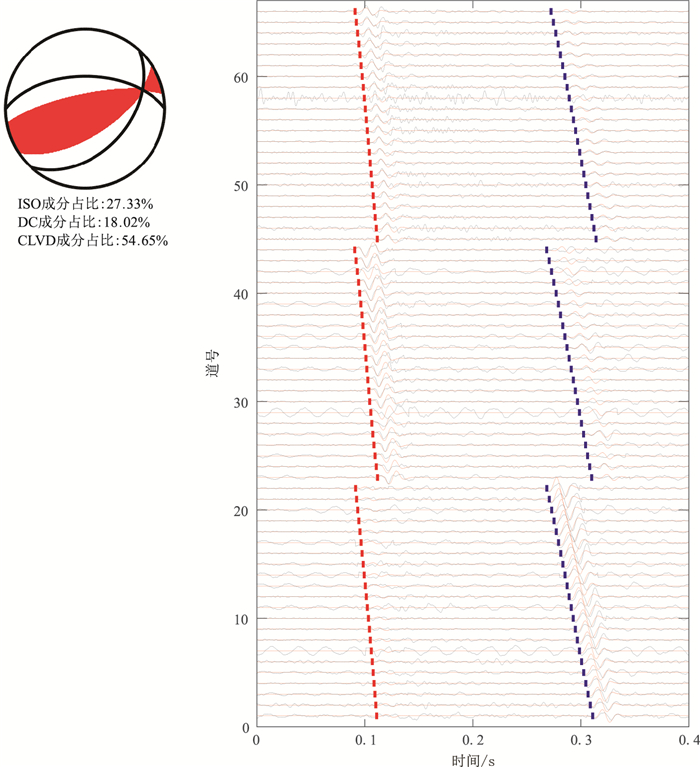图 12 井中观测第一个目标微地震事件震源机制反演结果和对应波形拟合 黑色实线表示实际数据波形，红色实线曲线表示反演结果的理论正演记录；红色和蓝色虚线分别表示手动拾取的纵波和横波初至。1~22道为x分量；23~44道为y分量；45~66道为z分量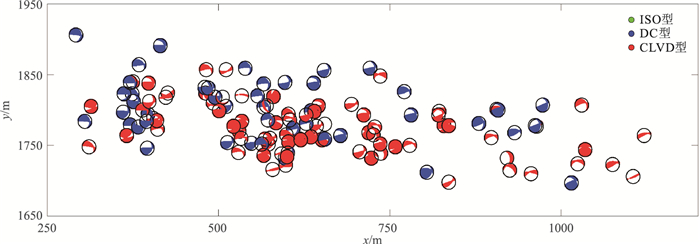图 13 123个目标微地震事件震源机制反演结果的沙滩球显示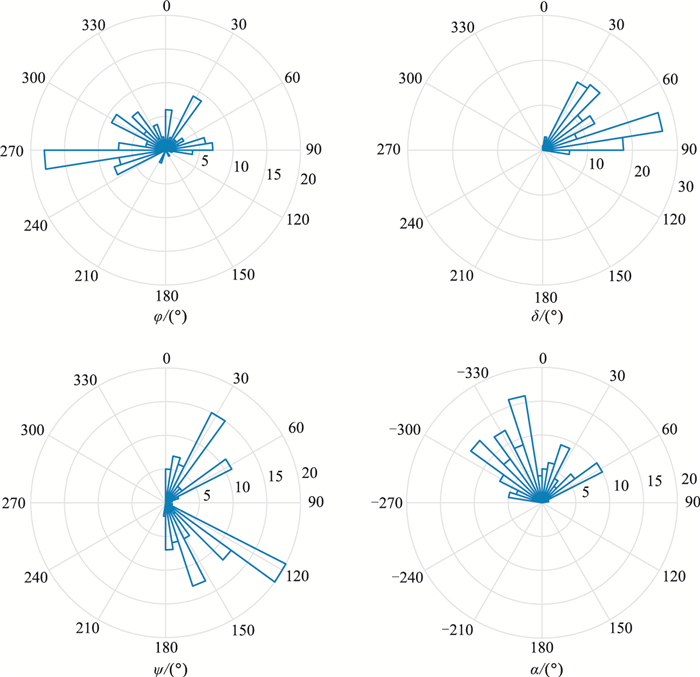图 14 微地震事件破裂面走向角、倾向角、滑动角及张裂角分布 扇形的长度表示事件个数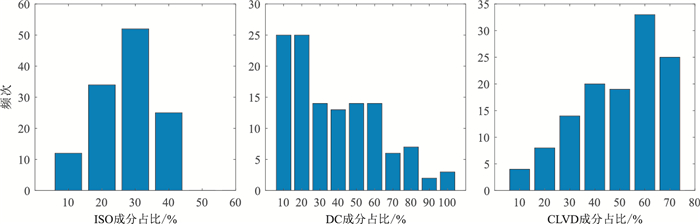图 15 微地震事件震源三种成分的占比统计直方图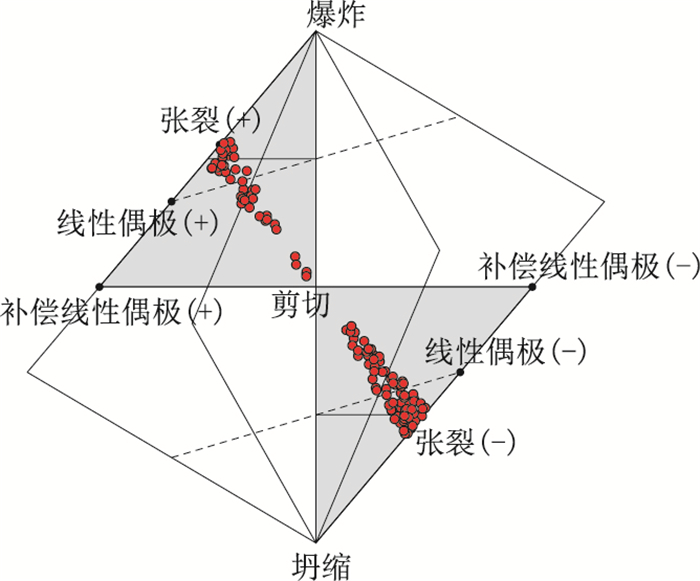图 16 实际微地震事件震源机制Hudson投影
4 结论

  Maxwell S C, Urbancic T I. The role of passive microseismic monitoring in the instrumented oil field[J]. The Leading Edge, 2001, 20(6): 636-639. DOI:10.1190/1.1439012  邹才能, 张国生, 杨智, 等. 非常规油气概念、特征、潜力及技术——兼论非常规油气地质学[J]. 石油勘探与开发, 2013, 40(4): 385-399. ZOU Caineng, ZHANG Guosheng, YANG Zhi, et al. Geological concepts, characteristics, resource potential and key techniques of unconventional hydrocarbon: On unconventional petroleum geology[J]. Petroleum Exploration and Development, 2013, 40(4): 385-399.  刘振武, 撒利明, 杨晓, 等. 页岩气勘探开发对地球物理技术的需求[J]. 石油地球物理勘探, 2011, 46(5): 810-818. LIU Zhenwu, SA Liming, YANG Xiao, et al. Needs of geophysical technologies for shale gas exploration[J]. Oil Geophysical Prospecting, 2011, 46(5): 810-818.  唐杰, 温雷, 王浩, 等. 正交各向异性介质中的剪张源震源机制与矩张量特征[J]. 石油地球物理勘探, 2018, 53(6): 1247-1255. TANG Jie, WEN Lei, WANG Hao, et al. Focal me-chanisms and moment tensor in orthorhombic anisotropic media[J]. Oil Geophysical Prospecting, 2018, 53(6): 1247-1255.  Pearson C. The relationship between microseismicity and high pore pressures during hydraulic stimulation experiments in low permeability granitic rocks[J]. Journal of Geophysical Research: Solid Earth, 1981, 86(B9): 7855-7864. DOI:10.1029/JB086iB09p07855  Phillips W S, Fairbanks T D, Rutledge J T, et al. Induced microearthquake patterns and oil-producing fracture systems in the Austin Chalk[J]. Tectonophysics, 1998, 289(1-3): 153-169. DOI:10.1016/S0040-1951(97)00313-2  Foulger G, Julian B, Hill D, et al. Non-double-couple microearthquakes at long valley caldera, California, provide evidence for hydraulic fracturing[J]. Journal of Volcanology Geothermal Research, 2004, 132(1): 45-71. DOI:10.1016/S0377-0273(03)00420-7  Šílenỳ J, Hill D P, Eisner L, et al. Non-double-couple mechanisms of microearthquakes induced by hydraulic fracturing[J]. Journal of Geophysical Research: Solid Earth, 2009, 114(B8): 1621-1643.  Vavryčuk V. Inversion for parameters of tensile earthquakes[J]. Journal of Geophysical Research: Solid Earth, 2001, 106(B8): 16339-16355. DOI:10.1029/2001JB000372  Aki K, Richards P G. Quantitative Seismology (Se-cond Edition)[M]. Sau-salito, California: University Science Books, 2002.  Vavryčuk V. On the retrieval of moment tensors from borehole data[J]. Geophysical Prospecting, 2007, 55(3): 381-391. DOI:10.1111/j.1365-2478.2007.00624.x  杨心超, 朱海波, 崔树果, 等. 纵波初动震源机制解在水力压裂微地震监测中的应用[J]. 石油物探, 2015, 54(1): 43-50. YANG Xinchao, ZHU Haibo, Cui Shuguo, et al. Application of P-wave first-motion focal mechanism solutions in microseismic monitoring for hydraulic fracturing[J]. Geophysical Prospecting for Petroleum, 2015, 54(1): 43-50. DOI:10.3969/j.issn.1000-1441.2015.01.006  杨心超, 朱海波, 李宏, 等. 基于纵波辐射花样的压裂微地震震源机制反演方法研究及应用[J]. 石油物探, 2016, 55(5): 640-648. YANG Xinchao, ZHU Haibo, LI Hong, et al. Microseismic focal mechanism inversion based on P-wave radiation pattern and its application[J]. Geophysical Prospecting for Petroleum, 2016, 55(5): 640-648. DOI:10.3969/j.issn.1000-1441.2016.05.002  翟鸿宇, 常旭, 王一博. 含衰减地层微地震震源机制反演及其反演分辨率[J]. 地球物理学报, 2016, 59(8): 3025-3036. ZHAI Hongyu, CHANG Xu, WANG Yibo, et al. Inversion for microseismic focal mechanisms in attenuated strata and its resolution[J]. Chinese Journal of Geophysics, 2016, 59(8): 3025-3036.  赵炜, 辛维, 毛中华, 等. 利用单井微地震波形能量反演震源机制[J]. 石油地球物理勘探, 2018, 53(5): 945-953. ZHAO Wei, XIN Wei, MAO Zhonghua, et al. Focal mechanism inversion with single-well microseismic wave energy[J]. Oil Geophysical Prospecting, 2018, 53(5): 945-953.  李晗, 姚振兴. 基于"剪切+张裂"一般位错模型频率域求解微震震源机制[J]. 地球物理学报, 2018, 61(3): 905-916. LI Han, YAO Zhenxing. Microseismic focal mechanism inversion in frequency domain based on general dislocation point model[J]. Chinese Journal of Geophysics, 2018, 61(3): 905-916.  李晗, 常旭. 微地震震源机制研究进展[J]. 中国科学: 地球科学, 2021, 51(3): 325-338. LI Han, CHANG Xun. A review of the microseismic focal mechanism research[J]. Science China: Earth Sciences, 2021, 51(3): 325-338.  唐杰, 温雷, 李聪, 等. 水力压裂诱发的剪张型微地震震源机制矩张量反演方法[J]. 石油地球物理勘探, 2019, 54(4): 826-835. TANG Jie, WEN Lei, LI Cong, et al. Hydraulic-fracturing shear-tensile microseismic focal mechanism moment tensor inversion[J]. Oil Geophysical Prospecting, 2019, 54(4): 826-835.  唐杰, 李聪, 刘英昌, 等. 剪张源约束的井中微地震震源机制反演方法[J]. 石油地球物理勘探, 2020, 55(1): 126-135. TANG Jie, LI Cong, LIU Yingchang, et al. Moment tensor inversion method from borehole data constrained by shear-tensile source model[J]. Oil Geophysical Prospecting, 2020, 55(1): 126-135.  谭玉阳, 胡隽, 张海江, 等. 利用全波形匹配方法确定水力压裂诱发地震震源机制[J]. 地球物理学报, 2019, 62(11): 4417-4436. TAN Yuyang, HU Jun, ZHANG Haijiang, et al. Source mechanism determination for hydraulic fracturing induced seismicity using full-waveform matching[J]. Chinese Journal of Geophysics, 2019, 62(11): 4417-4436. DOI:10.6038/cjg2019M0516  Gilbert F. Excitation of the normal modes of the Earth by earthquake sources[J]. Geophysical Journal International, 1971, 22(2): 223-226. DOI:10.1111/j.1365-246X.1971.tb03593.x  Vavryčuk V. Focal mechanisms in anisotropic media[J]. Geophysical Journal International, 2005, 161(2): 334-346. DOI:10.1111/j.1365-246X.2005.02585.x  Hudson J, Pearce R, Rogers R. Source type plot for inversion of the moment tensor[J]. Journal of Geophysical Research, 1989, 94(1): 765-774.  Stockwell R G, Mansinha L, Lowe R P. Localization of the complex spectrum: the S transform[J]. IEEE Transactions on Signal Processing, 1996, 44(4): 998-1001. DOI:10.1109/78.492555  王小杰, 印兴耀, 吴国忱. 基于S变换的吸收衰减技术在含气储层预测中的应用研究[J]. 石油物探, 2012, 51(1): 37-42. WANG Xiaojie, YIN Xingyao, WU Guochen. The application of an S transform based absorption and attenuation technique for prediction of gas-bearing re-servoir[J]. Geophysical Prospecting for Petroleum, 2012, 51(1): 37-42.  李波, 文晓涛, 张懿疆, 等. 基于Lucy-Richardson算法和广义S变换的Q值提取[J]. 石油物探, 2019, 58(5): 689-699. LI Bo, WEN Xiaotao, ZHANG Yijiang, et al. Combined generalized S transform and Lucy-Richardson algorithm for Q-value extraction[J]. Geophysical Prospecting for Petroleum, 2019, 58(5): 689-699. DOI:10.3969/j.issn.1000-1441.2019.05.007